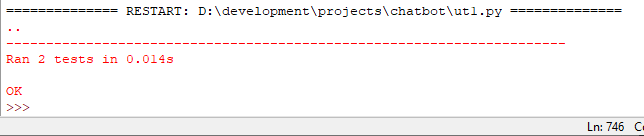# Part 2: Adding Structured Data to ChatBot

In the last article, I showed how one could build a chatbot and through brute force, get it to behave by calling different methods based on the prior question's input. Now, we want to use a data source to contain the questions and answers, then write a generic method that will retrieve the appropriate question and set of answers by the datasource.

#### Using a CSV (Comma Separated Value) file as the data source

Here, we are going to create a CSV file and read it into memory. We will use the rows to organize a given question and a corresponding set of answers for that question. Our method will 1) read the file into memory, 2) parse through each of the rows, 3) and then build a tuple of tuples (i.e. the first touple will contain the question paired with another tuple, which will be the set of answers for that question). We may even consider doing a tuple of tuple of tuples so that we can capture the indexes, but let's start simple and go more complicated.

Let's first create the CSV file and read it into memory. We can even assign the values to their corresponding tuples and print some samples. As before, we need to write the unit test case first, then we will write the method corresponding to that test.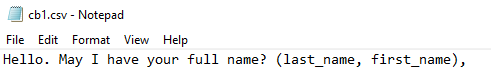Here's the basic unit test:

``````import unittest
import cb1

class TestChatbot1(unittest.TestCase):

response = cb1.get_start_question().split(".")
self.assertEqual(response.lower(), 'hello')

if __name__ == '__main__':
unittest.main()``````

Since we know that the first question is going to be "Hello. May I have your full name? (last_name, first_name),", we can simply test for the presence of the first word. I will split the first question into a list by the period (.) and take the first element in the list, which should be "Hello", then, I'll test the lower case format just to make sure that the test case and the file are case consistent.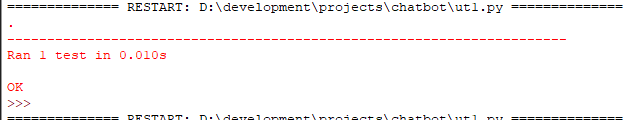The method will NOT be elegant yet. I want to take the time to step through each option, starting with the most basic and building on that to get something more efficient. So, let's start with reading a file (one line at a time), using Python's file reader and return just the first line in the file. We'll assume for now that the first line is the first question, although we will build a better approach later.

``````def read_file():

chatbotfile = 'cb1.csv'

with open(chatbotfile, 'r') as chatbotcontents:

return chatbotdata

def get_start_question():
return first_question

def main():
get_start_question()

if __name__ == '__main__':
main()``````

In this snippet, we are reading the CSV file into memory, where Python will add each line to a list. We then return the first element in the list as our first question. Our test case is testing for the first word, "Hello", and testing whether the first word of the first question is equal to "Hello".

This first question will take the user's name as input, therefore, there are no canned responses that we need to assign or include in the CSV, so, let's move onto the second question, which includes responses. I'll add the same question and responses from the prior article, which is as follows: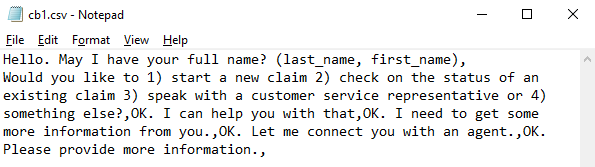Note: I had to remove the comma from the first question

We can now modify the test case to read the contents, however, we want to make some changes while we are reading the lines. Using the readlines method, Python will take each line and add it to a list, where each element of the list is one of the lines. We want to then iterate through that list to assign a new list for the question and each of the responses. So, in the end, it will look something like this:

[ ['question_1,], ['question_2', 'response_1', 'response_2',], [etc] ]

Our first test case will still select the first word of the first question, but we have to change it to get the first word of the line list within the list of lines, which should just be . Like so:

``````def test_filereader(self):
response = cb1.get_start_question().split(".")
self.assertEqual(response.lower(), 'hello')``````

In the code, we will create a first list of lines and then create a second empty list to contain the lines after we process each and covert the lines into lists of questions and responses.

``````def read_file():

chatbotfile = 'cb1.csv'

with open(chatbotfile, 'r') as chatbotcontents:

cb_lines = []
for line in chatbotdata:
line_list = line.split(",")
cb_lines.append(line_list)

return cb_lines``````

The code will now return a list of lists, where the master list is that of the lines, and the lists within it will be the sets of questions and responses. Let's test that everything is correct with the unit test: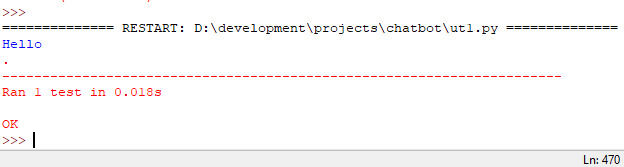If I just print the output, it looks like the following: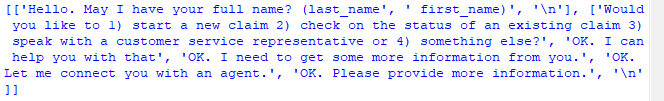Now for the magic. Let's write the code that will assign the questions and answers and interact with the user. We want to ask a question and capture a response, so let's write a method that will grab the line, ask the question, then assign the input to a variable. The test case should look exactly like the first article's test case:

class TestChatbot0(unittest.TestCase):

``````def test_firstnamelast(self):
self.assertEqual(cb0.question0("Dundas, Rob"), 'Thank you Rob.')

class TestChatbot1(unittest.TestCase):

def test_response1(self):
self.assertEqual(cb0.question1(1), 'OK. I can help you with that.')
def test_response2(self):
self.assertEqual(cb0.question1(2), 'OK. I need to get some more information from you.')
def test_response3(self):
self.assertEqual(cb0.question1(3), 'OK. Let me connect you with an agent.')
def test_response4(self):

``````

Now for the code...we're going to make a tweak to read the file into memory and then reference the in-memory file for each call to get a question:

``````
chatbotfile = 'cb1.csv'
with open(chatbotfile, 'r') as chatbotcontents:
cb_lines = []
for line in chatbotdata:
line_list = line.split(",")
cb_lines.append(line_list)
return cb_lines
def get_start_question(lines):
first_question = lines
return first_question
``````def get_first_response(lines):
first_response = lines
return first_response``````

Still very dumb and very labor intensive. As you can see, we could call this indefinitely, where we retrieve the question based on the row number. But, what if we added the response to the question fetch so that the response of the prior question would guide us to the fetch of the next question? Using the response from the question, we can add the response of the first question to the second question so that it will retrieve it dynamically. The list will look something like this (where q=question and r=response):

[[q1, r1], [q2,r1,r2-1,r2-1], [q3,r2,r3-1,r3-2]]

So, let's iterate through the rows and responses to check the rows for the response. Here's the Unit Test (note: I made a few tweaks after writing through the code this time because I realized that I wanted to keep the get_question and get_response methods for reading the line):

``````def test_question_from_response(self):
lines = cb1.read_file() # read the file
line = cb1.get_question_from_response(lines, "start question") # find line
q = cb1.get_question(line) # get question from response
r = cb1.get_response(line) # get response from response
name = "Rob"
response = r + " " + name # convert to answer
self.assertEqual(response.strip(), "Thank you Rob") # test``````

The code:

``````
def get_question_from_response(lines, response):
for line in lines:
if response == line:
return line
else:
pass
def get_question(line):
first_question = line
return first_question
``````def get_response(line):
first_response = line
return first_response``````

And running the test case: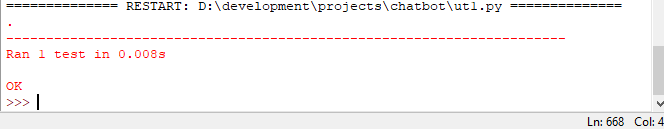So let's try for the second question using the dynamic approach. Note: we have to add the first response to the second question, second item in the list. So, the CSV now looks like: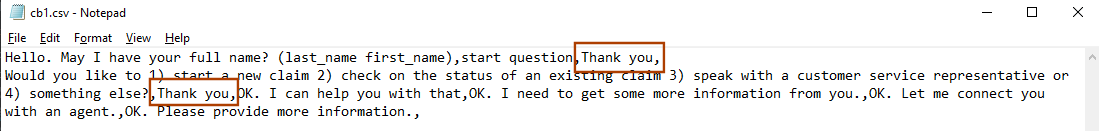The test case:

``````def test_second_question_from_response(self):
lines = cb1.read_file() # read the file
line1 = cb1.get_question_from_response(lines, "start question") # find line
line2 = cb1.get_question_from_response(lines, cb1.get_response(line1))
q2 = cb1.get_question(line2)
r2 = cb1.get_response(line2)
first_phrase = q2.split(")")
self.assertEqual(first_phrase, "Would you like to 1")``````

The code:

``````def get_question_from_response(lines, response):

for line in lines:
if response == line:
return line
else:
pass

def get_question(line):
first_question = line
return first_question

def get_response(line):
first_response = line
return first_response``````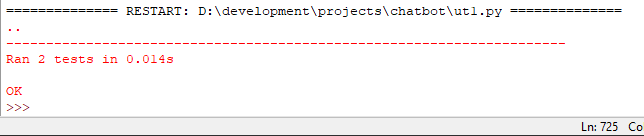Before leaving this concept, Python includes a CSV reader, so let's incorporate the CSV reader to see if it can simplify the code at all. We'll leave the test cases the same, but change the methods to read and use the CSV package instead of the file reader.

I'm not sure that this saved any time for this, but it does allow the csv to be changed without having to re-write the code. For example, we can add more columns and as long as the field names stay the same, we should be OK:

``````import csv

chatbotfile = 'cb1.csv'

with open(chatbotfile, newline='') as csvfile: